# Overview¶

The most fundamental, basic analysis of economics is supply and demand. We'll use this post as an opportunity to review the basics of supply and demand as well as explore plotting techniques in Python.

If you want to understand supply and demand, you have to keep in mind that perspective is everything. I am a consumer, so from my point of view my interests are represented by the demand curve. I will demand a higher quantity of goods if the prices is lower (capital is scarce, so I want the most stuff for the least money). On the other side of the coin are businesses who will supply a higher quantity if they can charge higher prices.

You can feel already (and you will see visually below) that these two forces run in different directions. Moreover, these two processes depend on each other. Demand affects supply and supply affects demand (to use a fancy word, they are endogenous). When these two opposite yet dependent forces balance it is called equilibrium.

In :
# load libraries
from matplotlib import pyplot as plt
import numpy as np


# Demand¶

In :
# demand function
def demand(x):
return -x + 10

plt.xkcd() # xkcdify our graphs

data = np.arange(1, 10, 1) # use numpy to create a range of data

plt.plot(data, demand(data), 'dodgerblue', linewidth=5.0) #create the plot

plt.axis((0,10,0,10)) # set axis limits

plt.xticks([]) # remove ticks
plt.yticks([]) # remove ticks

plt.xlabel("Quantity") # label the axes
plt.ylabel("Price") # label the axes

plt.annotate('Demand', xy=(1.2,8.5)) # annotate the graph

plt.show() # display the graph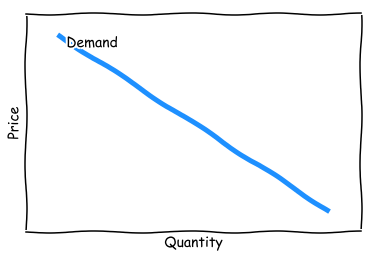The most important aspect of the demand curve is the negative slope: the quantity demanded rises when price falls. The reverse is also true: as price rises, quantity demanded falls. Remember that perspective is key. Are you looking at the curve from the perspective of price or quantity? Are you a consumer or a supplier?

## Shift in Demand¶

In :
# to plot the shift in demand graph, we simply add a shifted function
# most of the code is the same

# demand1 function
def d1(x):
return -x + 10

# demand2 function
def d2(x):
return -x + 5

plt.xkcd() # xkcdify our graphs

d1_data = np.arange(5, 10, 1) # produce data for the graphs
d2_data = np.arange(1, 6, 1) # produce data for the graphs

plt.plot(d1_data, d1(d1_data), "dodgerblue", d2_data, d2(d2_data), "red", linewidth=5.0)

plt.axis((0,10,-2,7)) # axis range

plt.xticks([]) # remove ticks
plt.yticks([]) # remove ticks

plt.xlabel("Quantity") # label the axes
plt.ylabel("Price") # label the axes

plt.annotate("D1", xy=(5.1,5))
plt.annotate("D2", xy=(1.2,3.9))

# we can add an arrow that points to the xy coors specified
plt.annotate("Decrease\nin Demand", xy=(2.9,2.3), arrowprops=dict(arrowstyle='->'), xytext=(5,2))

plt.show()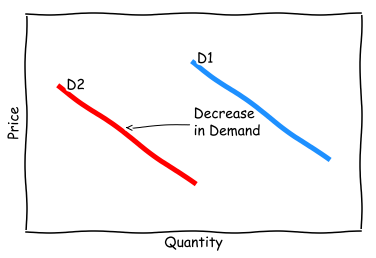The demand curve is not an eternal relationship; it can change over time. Demand for cigarettes, for example, fell dramatically after medical warnings about their ill effects. For a time, the price remained the same, but the quantity demanded fell (represented by the curve moving towards zero on the x axis).

# Supply¶

In :
# supply function
def supply(x):
return x

plt.xkcd() # xkcdify our graphs

supp = plt.plot(data, supply(data))

plt.axis((0,10,0,10)) # axis range

plt.xticks([]) # remove ticks
plt.yticks([]) # remove ticks

plt.setp(supp, color='limegreen', linewidth=5.0)

plt.xlabel("Quantity") # label the axes
plt.ylabel("Price") # label the axes

plt.annotate('Supply', xy=(1.8,1.5))

plt.show()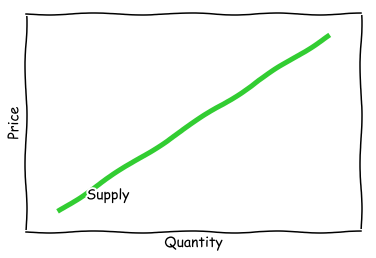The supply curve has positive slope - the opposite of the demand curve. The higher the price of something, the more quantity will be provided. The relationship between price and quantity in the supply curve played an integral role the debate over price gouging during the aftermath of hurricane Charley. As Michael Sandel relates in his book Justice, officials were hesitant to limit price gouging because, theoretically, the higher price would attract a higher quantity of supplies to devastated regions. Uber's 'surge pricing' operates under the same premise.

## Supply Curve Shift¶

In :
# supply1 function
def s1(x):
return x

# supply2 function
def s2(x):
return x+5

# plot demand function
plt.xkcd()

s1_data = np.arange(5, 10, 1) # produce data for the graphs
s2_data = np.arange(1, 6, 1) # produce data for the graphs

plt.plot(s1_data, s1(s1_data), "red", s2_data, s2(s2_data), "limegreen", linewidth=5.0)

plt.axis((0,10,2,12)) # axis range

plt.xticks([]) # remove ticks
plt.yticks([]) # remove ticks

plt.xlabel("Quantity") # label the axes
plt.ylabel("Price") # label the axes

plt.annotate("S1", xy=(1,5.4))
plt.annotate("S2", xy=(5.4,4.8))
plt.annotate("Increase\nin Supply", xy=(5.5,6), arrowprops=dict(arrowstyle='->'), xytext=(2,5.4))

plt.show()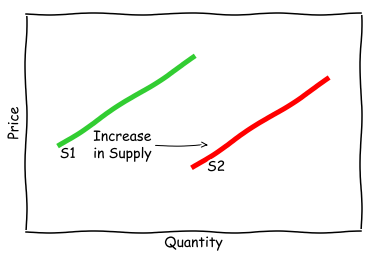Just like demand, the supply curve can also shift. Here we see an increase in supply for every price. This can occur if the inputs into production fall in cost, therefore making each unit more profitable. Because of the increase in profit per unit, suppliers will produce a higher quantity for the same price.

# Equilibrium¶

In :
plt.xkcd() # xkcdify our graphs

plt.plot(data, demand(data), 'dodgerblue', data, supply(data), 'limegreen', linewidth=5.0)

plt.axis((0,10,0,10)) # axis range

plt.xticks([]) # remove ticks
plt.yticks([]) # remove ticks

plt.xlabel("Quantity") # label the axes
plt.ylabel("Price") # label the axes

plt.annotate('Equilibrium', xy=(5,5))
plt.annotate('Demand', xy=(1.5,8.5))
plt.annotate('Supply', xy=(1.8,1.5))

plt.show()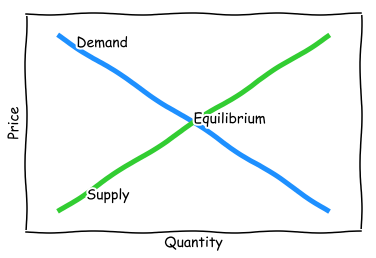Equilibrium occurs when the price and quantity demanded matches the price and quantity supplied. The market will naturally tend towards to equilibrium as suppliers and consumers seek greater efficiencies in the deployment of resources. Mismatches in supply and demand will lead to surpluses and shortages (depending on your perspective).

# Expected Return vs Required Return¶

Expected return and required return are fundamental concepts of finance. One of the clearest ways to understand these two concepts is with supply and demand. Antti Ilmanen in Expected Returns says,

The expected return determines how high a return the market can feasibly supply, while the required return determines how high a return market participants (as a group) demand... As always, market prices adjust to balance supply and demand. For example, if all investors suddenly require higher risk premia, current market prices of risky assets must fall.

The balancing between expected and required returns is one of the core assumptions of the capital asset pricing model. One reason why CAPM fails is because of irrational pricing that distorts the market equilibrium.